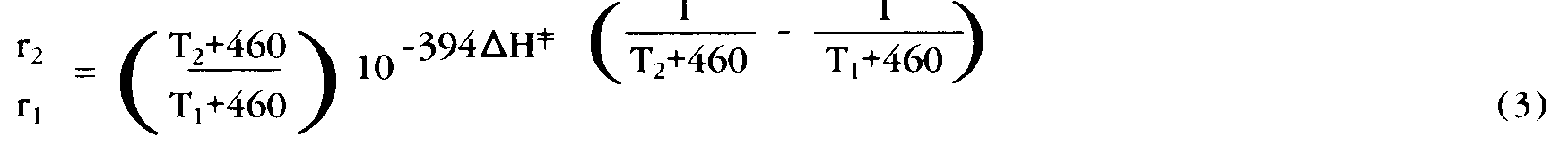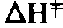# Isoperms–Temperature

It is widely recognized that most chemical reactions proceed at a faster
rate as the temperature is increased. Many of us are familiar with the
rule of thumb stated in elementary chemistry courses that “chemical reaction
rates double with each 10 degrees C increase in temperature.” However,
in truth, each reaction displays a different specific “sensitivity” to
temperature changes increasing by more or less than the factor of 2. Physical
chemists have developed a theory of chemical reaction rates (Transition

It is widely recognized that most chemical reactions proceed at a faster rate as the temperature is increased. Many of us are familiar with the rule of thumb stated in elementary chemistry courses that “chemical reaction rates double with each 10 degrees C increase in temperature.” However, in truth, each reaction displays a different specific “sensitivity” to temperature changes increasing by more or less than the factor of 2. Physical chemists have developed a theory of chemical reaction rates (Transition State Theory) that can accurately describe the effect of temperature changes on reaction rates. Their equation for the relative rate of reaction at two (Fahrenheit) temperatures T1 and T2

Erratum:In the following formula, the printed text contains an error. For

```    r2

r1

r2

--

r1
```
``````

incorporates a constant, the enthalpy of activation,, a thermodynamic function that quantifies the sensitivity of a specific reaction to temperature changes. ;has a specific value that may be experimentally measured for each paper. Most (of a limited number) of such experimental measurements place; in the range of 20-35 Kcal (kilocalorie) with a value of 30-35 Kcal providing a very good approximation for most preservation applications.

Up to this point we have examined the effects of changing temperature and percent relative humidity individually in both qualitative and quantitative terms. What remains is to combine their effects into a single equation that will be transformed into the graphical isoperm formulation.